# 24.3 Matrices in graphics

#### Attitude

Now let's take a more detailed look at the Matrix Multiply step of the graphics pipeline. We'll discuss the more general three-dimensional case.

In the Matrix Multiply step we right-multiply our input vertices by a series of three matrices, first the model matrix, second the view matrix, and third the projection matrix. Although we list the matrices from left to right in our formulas, and in fact feed them into the graphics pipeline in the left-to-right order, the matrices act on the vertices in the reverse right-to-left order. In other words, the matrix closest to the vertex in the ordering of the formula acts on the vertex first.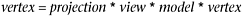The model matrix is frequently just the cMatrix _attitude member of the cCritter the vertex belongs to. The _attitude specifies a critter's orientation and its location. We think of each critter as having its own attitude trihedron , meaning a set of three mutually perpendicular unit vectors. As the critter pitches, yaws, and rolls, the vectors of its attitude trihedron move. At every step of the update we are careful to ensure that the trihedron vectors remain mutually perpendicular and of unit length. As the critter moves about, its position changes as well. A critter's attitude embodies both its trihedron information and its position information. In Figure 24.4 we show not only a critter with its attitude in a 3D world, but also a viewer critter that will be used for projecting the 3D world to 2D plane that can be mapped to an onscreen window. We will take into account the viewer critter's attitude as well.

##### Figure 24.4. A critter's attitude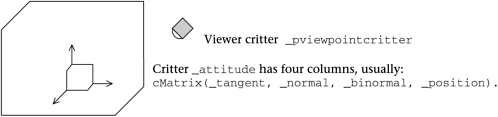In the Pop Framework, the cVector class represents three-dimensional vectors, and the cMatrix class represents the matrices used for our graphics. We think of our vectors as being column matrices of size three by one. Although you might expect the matrices to be three rows by three columns , they are actually three by four, that is, they have an extra column. This is a standard computer graphics trick.

The matrices are three by four in size so that we can use the first three columns to represent rotations and the fourth column to represent translations. We might say that one of our 3D graphics matrices M is really a square three by three matrix R and an extra column T, that is, we could informally say M = ( R , T ). If V is a vector, we compute M * V as R * V + T , where the R * V computation is done in the same way that we would always multiply a square three by three matrix times a three by one column matrix.

Mathematically we can make the computation seem consistent by imagining that the matrix has a fourth row holding 0, 0, 0, 1, and that the vector has a fourth row holding 1.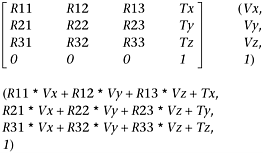By default, we match the x -, y -, and z -axes of a critter's attitude matrix to, respectively, the critter's cVector _tangent , _normal , and _binormal variables . The tangent always points in the direction the critter is moving (or has most recently moved). The normal points in the direction in which the critter is turning (or has most recently turned). The binormal is the cross product (tangent * normal), a vector perpendicular to the first two. That is, by default we keep updating our critter's attitude matrix to have the following form.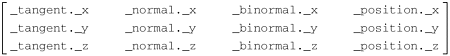Doing this makes the critters have a pleasing kind of motion, as they move about; they turn and roll like birds or fish. Sometimes, however, you may want to keep a strict control over your critter's attitude. In this case, you must use the cCritter::setAttitudeToMotionLock method to set the cCritter _attitudetomotionlock field to FALSE in place of the default TRUE .

Spacewar game critters with their attitudes locked to their motions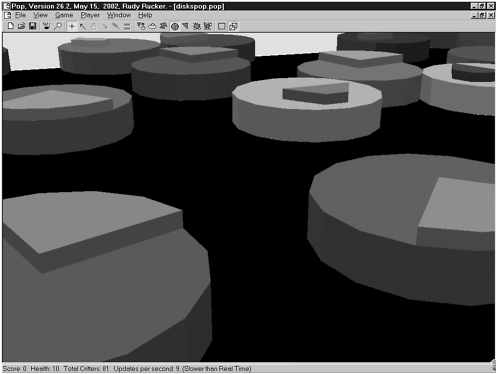A further complication arises in the case where the critter has a sprite that's offset or rotated from the critter's location and orientation. In order to manage this, we give the cSprite class a _spriteattitude field. When we feed a critter's model matrix into the graphics pipeline, we actually feed in attitude() * spriteattitude() .

#### The view matrix

Let's look at our 3D graphics pipeline's Matrix Multiply step again.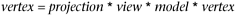The purpose of the view transformation is to show the world as seen by the viewer critter. The original coordinates of the critter are given in terms of the world coordinate system. The view transformation is designed to change from the world coordinates to the viewer coordinates. Although the viewer is out at some arbitrary position, the viewer thinks of itself as being at the origin of its own coordinate system. Although the viewer may be pitched, yawed, and rolled relative to the world, the viewer imagines itself to be at the center of its own standard-oriented coordinate system. Although the world thinks of the critter as having a fairly arbitrary viewpointcritterattitude , the viewer thinks of its attitude as being the identity matrix cMatrix::IDENTITY . In Figure 24.5 we show the viewer critter's attitude. The viewer critter is going to think of its position as the origin of a coordinate system, and its attitude vectors as the x -, y -, and z -axes.

##### Figure 24.5. The viewer critter's attitude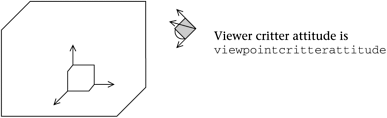The remarks about the viewer thinking it's at the center of things can be represented by an equation.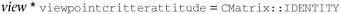Assume that we have a cMatrix::inverse() operation to compute the multiplicative inverse of a matrix; this is analogous to the way that the multiplicative inverse of, say, 2 is 0.5. Then we can rewrite our equation like this.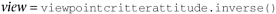This is one of the little miracles of computer graphics. The tedious matrix inverse operations have a use!

An interesting viewpoint for the PickNPop game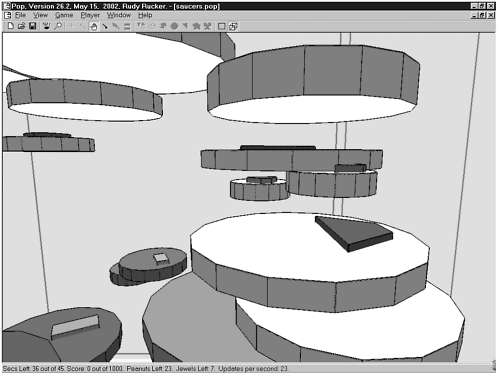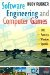Software Engineering and Computer Games
ISBN: B00406LVDU
EAN: N/A
Year: 2002
Pages: 272